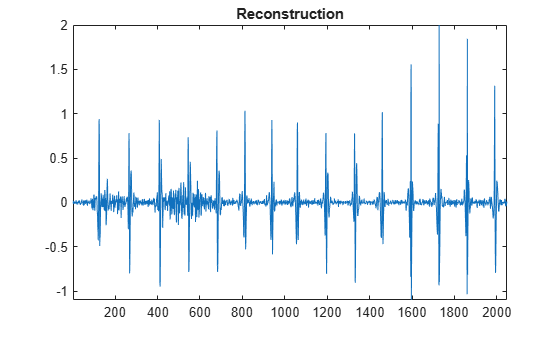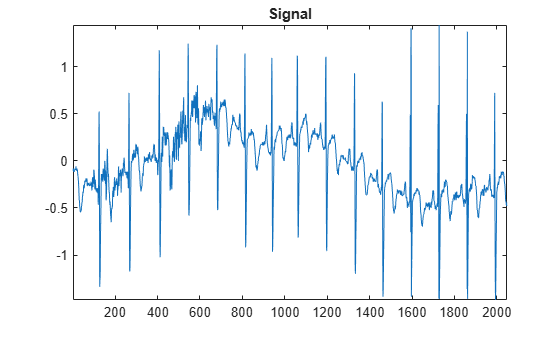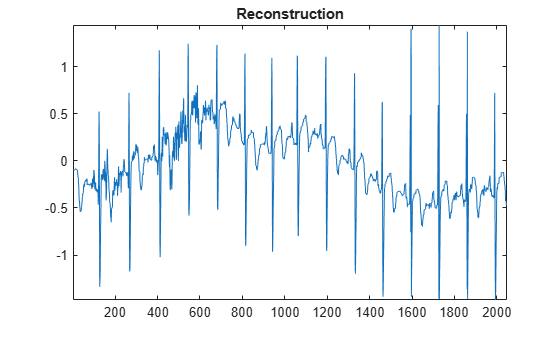# wthcoef

1-D wavelet coefficient thresholding

## Syntax

``nc = wthcoef("a",c,l)``
``nc = wthcoef("d",c,l,n)``
``nc = wthcoef("d",c,l,n,p)``
``nc = wthcoef("t",c,l,n,t,sorh)``

## Description

`wthcoef` thresholds wavelet coefficients for the denoising or compression of a 1-D signal.

example

````nc = wthcoef("a",c,l)` returns coefficients obtained from the multilevel wavelet decomposition structure [`c`,`l`] by setting the approximation coefficients to zero . For information about the decomposition structure, see `wavedec`.```
````nc = wthcoef("d",c,l,n)` returns coefficients obtained from [`c`,`l`] by setting all the coefficients at detail levels specified in `n` to zero.```

example

````nc = wthcoef("d",c,l,n,p)` returns coefficients obtained from [`c`,`l`] by rate compression defined in vectors `n` and `p`. `n` specifies the detail levels to be compressed and `p` the corresponding percentages of lower coefficients to set to zero. `n` and `p` must be of the same length.```
````nc = wthcoef("t",c,l,n,t,sorh)` returns coefficients obtained from [`c`,`l`] by thresholding specified in `thr`. `n` specifies the detail levels to be thresholded and `t` the corresponding thresholds. `n` and `t` must be of the same length.```

## Examples

collapse all

Load and plot a 1-D signal.

```load wecg plot(wecg) title("Signal") axis tight```Obtain a level 3 wavelet decomposition of the signal using the Daubechies `db4` wavelet.

```wv = "db4"; [c,l] = wavedec(wecg,3,wv);```

Use `wthcoef` to modify the wavelet decomposition `c`. Set the approximation coefficients to zero and plot the difference between the original and modified wavelet decompositions.

```nc = wthcoef("a",c,l); plot(c-nc) title("Difference") axis tight```Reconstruct a signal using the modified wavelet decomposition.

```xrec = waverec(nc,l,wv); plot(xrec) title("Reconstruction") axis tight```Save the current extension mode. Change the extension mode to periodized extension.

```origmode = dwtmode("status","nodisplay"); dwtmode("per","nodisplay")```

Load and plot a 1-D signal. The signal has 2048 samples.

```load wecg plot(wecg) title("Signal") axis tight```Obtain a level 2 wavelet decomposition of the signal using the Haar wavelet. Display the bookkeeping vector. Confirm there are 1024 detail coefficients at level 1, and 512 detail coefficients at level 2.

```wv = "haar"; [c,l] = wavedec(wecg,2,wv); l```
```l = 4×1 512 512 1024 2048 ```

Use `wthcoef` to threshold the level 1 and level 2 detail coefficients in the wavelet decomposition `c`. Set 75% of the level 1 detail coefficients to zero, and set 50% of the level 2 detail coefficients to zero.

`nc = wthcoef("d",c,l,[1 2],[75 50]);`

Confirm there are 256 nonzero wavelet coefficients at levels 1 and 2 in the modified wavelet decomposition `nc`.

```[level1,level2] = detcoef(nc,l,[1 2]); [nnz(level1) 1024*0.25]```
```ans = 1×2 256 256 ```
`[nnz(level2) 512*0.5]`
```ans = 1×2 256 256 ```

Reconstruct a signal using the modified wavelet decomposition.

```xrec = waverec(nc,l,wv); plot(xrec) title("Reconstruction") axis tight```Restore the original extension mode.

`dwtmode(origmode,"nodisplay")`

## Input Arguments

collapse all

Wavelet decomposition, specified as a vector. The vector contains the wavelet coefficients. The bookkeeping vector `l` contains the number of coefficients by level. `c` is the output of `wavedec`.

Data Types: `single` | `double`
Complex Number Support: Yes

Bookkeeping vector, specified as a vector of positive integers. The bookkeeping vector is used to parse the coefficients in the wavelet decomposition `c` by level. `l` is the output of `wavedec`.

Data Types: `single` | `double`

Detail levels, specified as a vector of positive integers less than or equal to `N`, where `N` is the level of the wavelet decomposition used to obtain [`c`,`l`]. Specifically, `N = length(l)-2`.

Data Types: `double`

Percentages of coefficients to set to zero, specified as a vector of positive integers less than or equal to 100. `p` and `n` must be the same length.

Data Types: `double`

Thresholds to apply to detail coefficients, specified as a real-valued vector. `t` and `n` must be the same length.

Data Types: `double`

Type of thresholding to perform:

• `"s"` — Soft thresholding

• `"h"` — Hard thresholding

## Output Arguments

collapse all

Modified wavelet decomposition, returned as a vector. `nc` and `c` have equal length.

## Version History

Introduced before R2006a